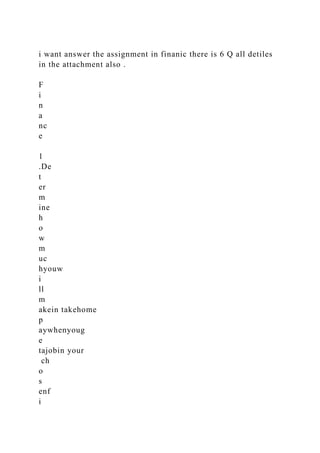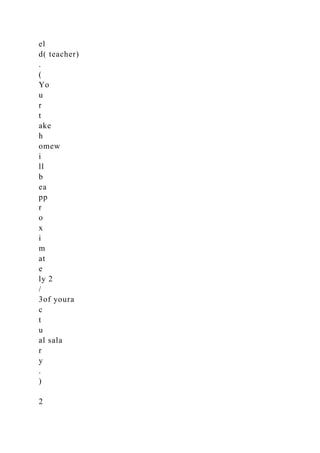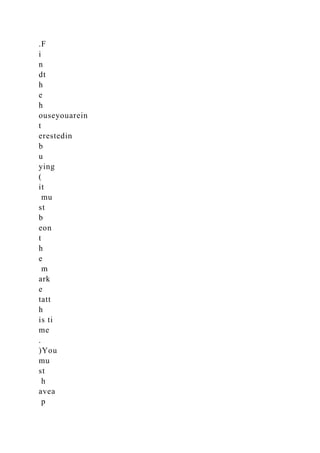Seu SlideShare está sendo baixado. ×

# i want answer the assignment in finanic there is 6 Q all detiles in .docx

Anúncio
Anúncio
Anúncio
Anúncio
Anúncio
Anúncio
Anúncio
Anúncio
Anúncio
Anúncio
AnúncioAnúncio
Anúncio
Carregando em…3
×

1 de 11 Anúncio

# i want answer the assignment in finanic there is 6 Q all detiles in .docx

i want answer the assignment in finanic there is 6 Q all detiles in the attachment also .

F
i
n
a
nc
e

1
.De
t
er
m
ine
h
o
w
m
uc
hyouw
i
ll
m
akein takehome
p
aywhenyoug
e
tajobin your
ch
o
s
enf
i
el
d( teacher)
.
(
Yo
u
r
t
ake
h
omew
i
ll
b
ea
pp
r
o
x
i
m
at
e
ly 2
/
3of youra
c
t
u
al sala
r
y
.
)

2
.F
i
n
dt
h
e
h
ouseyouarein
t
erestedin
b
u
ying
(
it
mu
st
b
eon
t
h
e
m
ark
e
tatt
h
is ti
me
.
)You
mu
st
h
avea
p
i
c
t
u
reof t
h
e
h
ou
s
e,a
d
es
c
rip
t
i
o
nof the
h
o
u
sea
n
d
p
roper
t
y,a
n
dt
h
n
g
p
riceof t
h
e pro
p
erty.

3.Statet
h
eit
em
s
th
atyoulikea
n
d
d
is
l
ikea
b
outt
h
e
h
ouseor
p
r
o
p
erty.

4.F
i
n
dyour
m
ont
h
ly
p
ay
m
e
n
tsif you
p
ay2
0
%
d
o
w
na
n
dta
k
e
o
u
ta
m
o
r
tga
g
ef
o
r
30years
a
t5.6%. F
i
n
dt
h
e t
o
tala
m
ou
n
t
y
ouw
i
ll
h
ave
p
aidt
h
e ba
n
kat
t
h
eendof t
h
e30years.

5.F
i
n
dyour
m
ont
h
ly
p
ay
m
e
n
tsif you
p
ay2
0
%
d
o
w
na
n
dta
k
e
o
u
ta
m
o
r
tga
g
ef
o
r
1
5years
a
t5.6%. F
i
n
dt
h
e t
o
tala
m
ou
n
t
y
ouw
i
ll
h
ave
p
a
idt
h
e ba
n
kat
t
h
eendof t
h
e15years.

6.F
i
n
dy
o
u
r
m
ont
h
ly
p
ay
m
e
n
tsifyou
p
ay10%
d
o
w
na
n
dta
k
e
o
u
ta
m
o
r
tga
g
ef
o
r

30years
a
t5.6%. F
i
n
dt
h
e t
o
tala
m
ou
n
t
y
ouw
i
ll
h
ave
p
aidt
h
e ba
n
kat
t
h
eendof t
h
e30years.
.

i want answer the assignment in finanic there is 6 Q all detiles in the attachment also .

F
i
n
a
nc
e

1
.De
t
er
m
ine
h
o
w
m
uc
hyouw
i
ll
m
akein takehome
p
aywhenyoug
e
tajobin your
ch
o
s
enf
i
el
d( teacher)
.
(
Yo
u
r
t
ake
h
omew
i
ll
b
ea
pp
r
o
x
i
m
at
e
ly 2
/
3of youra
c
t
u
al sala
r
y
.
)

2
.F
i
n
dt
h
e
h
ouseyouarein
t
erestedin
b
u
ying
(
it
mu
st
b
eon
t
h
e
m
ark
e
tatt
h
is ti
me
.
)You
mu
st
h
avea
p
i
c
t
u
reof t
h
e
h
ou
s
e,a
d
es
c
rip
t
i
o
nof the
h
o
u
sea
n
d
p
roper
t
y,a
n
dt
h
n
g
p
riceof t
h
e pro
p
erty.

3.Statet
h
eit
em
s
th
atyoulikea
n
d
d
is
l
ikea
b
outt
h
e
h
ouseor
p
r
o
p
erty.

4.F
i
n
dyour
m
ont
h
ly
p
ay
m
e
n
tsif you
p
ay2
0
%
d
o
w
na
n
dta
k
e
o
u
ta
m
o
r
tga
g
ef
o
r
30years
a
t5.6%. F
i
n
dt
h
e t
o
tala
m
ou
n
t
y
ouw
i
ll
h
ave
p
aidt
h
e ba
n
kat
t
h
eendof t
h
e30years.

5.F
i
n
dyour
m
ont
h
ly
p
ay
m
e
n
tsif you
p
ay2
0
%
d
o
w
na
n
dta
k
e
o
u
ta
m
o
r
tga
g
ef
o
r
1
5years
a
t5.6%. F
i
n
dt
h
e t
o
tala
m
ou
n
t
y
ouw
i
ll
h
ave
p
a
idt
h
e ba
n
kat
t
h
eendof t
h
e15years.

6.F
i
n
dy
o
u
r
m
ont
h
ly
p
ay
m
e
n
tsifyou
p
ay10%
d
o
w
na
n
dta
k
e
o
u
ta
m
o
r
tga
g
ef
o
r

30years
a
t5.6%. F
i
n
dt
h
e t
o
tala
m
ou
n
t
y
ouw
i
ll
h
ave
p
aidt
h
e ba
n
kat
t
h
eendof t
h
e30years.
.

Anúncio
Anúncio

### i want answer the assignment in finanic there is 6 Q all detiles in .docx

1. 1. i want answer the assignment in finanic there is 6 Q all detiles in the attachment also . F i n a nc e 1 .De t er m ine h o w m uc hyouw i ll m akein takehome p aywhenyoug e tajobin your ch o s enf i
2. 2. el d( teacher) . ( Yo u r t ake h omew i ll b ea pp r o x i m at e ly 2 / 3of youra c t u al sala r y . ) 2
3. 3. .F i n dt h e h ouseyouarein t erestedin b u ying ( it mu st b eon t h e m ark e tatt h is ti me . )You mu st h avea p
4. 4. i c t u reof t h e h ou s e,a d es c rip t i o nof the h o u sea n d p roper t y,a n dt h e aski n g p
5. 5. riceof t h e pro p erty. 3.Statet h eit em s th atyoulikea n d d is l ikea b outt h e h ouseor p r o p erty. 4.F i n dyour m
6. 6. ont h ly p ay m e n tsif you p ay2 0 % d o w na n dta k e o u ta m o r tga g ef o r 30years a t5.6%. F i
7. 7. n dt h e t o tala m ou n t y ouw i ll h ave p aidt h e ba n kat t h eendof t h e30years. 5.F i n dyour m ont h ly
8. 8. p ay m e n tsif you p ay2 0 % d o w na n dta k e o u ta m o r tga g ef o r 1 5years a t5.6%. F i n dt
9. 9. h e t o tala m ou n t y ouw i ll h ave p a idt h e ba n kat t h eendof t h e15years. 6.F i n dy o u r m ont
10. 10. h ly p ay m e n tsifyou p ay10% d o w na n dta k e o u ta m o r tga g ef o r 30years a t5.6%. F i n dt
11. 11. h e t o tala m ou n t y ouw i ll h ave p aidt h e ba n kat t h eendof t h e30years.